# Fitness Equipment

Grid List\$3249.00
Increase value Decrease value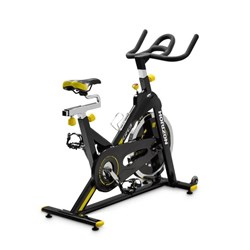\$799.00
Increase value Decrease value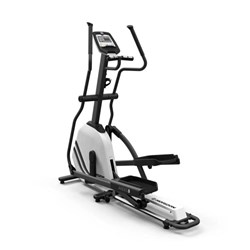\$1698.99
Increase value Decrease value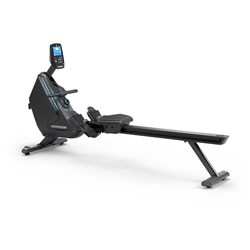\$1698.99
Increase value Decrease value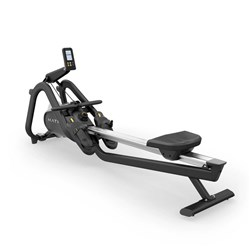Matrix Rower
\$2299.00
Increase value Decrease value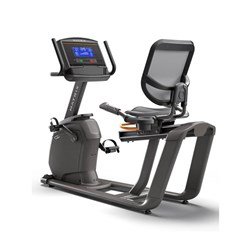\$3199.00
Increase value Decrease value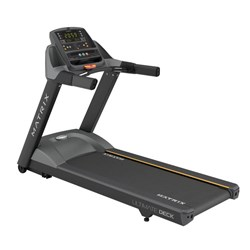\$5299.00
Increase value Decrease value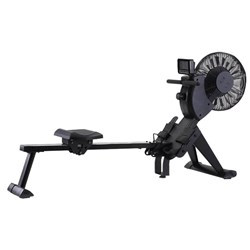Tempo Air Rower
\$699.00
Increase value Decrease value\$3099.00
Increase value Decrease value
\$368.50
Increase value Decrease value
\$5390.00
Increase value Decrease value
Fitness Reformer
\$3799.00
Increase value Decrease value
\$5174.00
Increase value Decrease value
\$338.00
Increase value Decrease value
\$5695.00
Increase value Decrease value
\$5173.99
Increase value Decrease value
\$2099.00
Increase value Decrease value
Reformer Box
\$367.99
Increase value Decrease value
Thoracic Barrel
\$474.99
Increase value Decrease value
\$7899.00
Increase value Decrease value
\$8699.00
Increase value Decrease value
\$1999.00
Increase value Decrease value
P.O.A.
P.O.A.
P.O.A.
P.O.A.
P.O.A.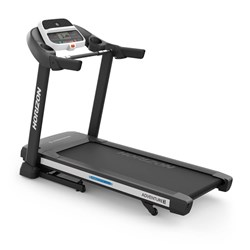\$2099.00
Increase value Decrease value
\$2399.00
Increase value Decrease value
\$3298.90
Increase value Decrease value
Grid List
##### Products to compare:
Comparing Products